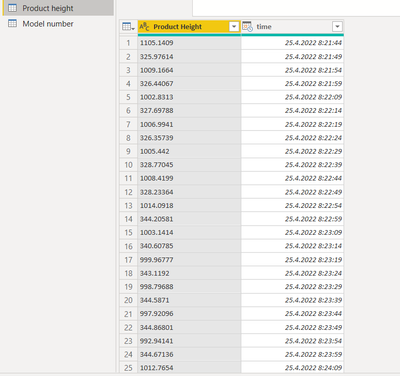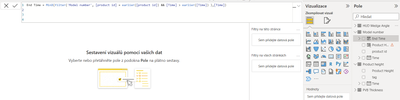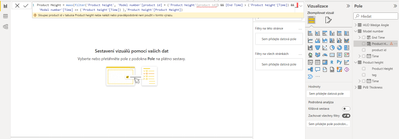cancel
Showing results for
Did you mean:

Fabric is Generally Available. Browse Fabric Presentations. Work towards your Fabric certification with the Cloud Skills Challenge.Frequent Visitor

## Merge product id based on time range/timestamps from another table

Hello all,

I am trying to figure out how to merge product ID based on time ranges from another tables.

I have 4 tables. One table consists of Product IDs and timestamps when the specific product was produced. So for example product ID 173 was produced from 25. 4. 2022 8:08:04 until 25.4.200 11:29:30 when the Product ID 336 started.In the other tables I have some metrics measurements, their values and timestamps which are updating every 5 secs. So all of these timestamps should have Product ID 173 based on the table above.What I want to solve is how to recognize the product ID based on the time ranges in the measurements tables. Is it somehow possible to realize?
Thank you so much for your help.

1 ACCEPTED SOLUTIONCommunity Support

You could add two calculated columns to get the start and end time for a [Product ID] on Model number table:

``````start_ =
CALCULATE (
MIN ( 'model number'[time] ),
FILTER (
'model number',
'model number'[product ID] = EARLIER ( 'model number'[product ID] )
)
)
``````
``````end_ =
CALCULATE (
MAX ( 'model number'[time] ),
FILTER (
'model number',
'model number'[product ID] = EARLIER ( 'model number'[product ID] )
)
)
``````

Then add [product ID] to the Product height table:

``````Product_ID =
CALCULATE (
MAX ( 'model number'[Product ID] ),
FILTER (
'model number',
'Product height'[time] >= 'model number'[start_]
&& 'Product height'[time] <= 'model number'[end_]
)
)
``````

Best Regards,

Jay

Community Support Team _ Jay
If this post helps, then please consider Accept it as the solution
to help the other members find it.
3 REPLIES 3Community Support

You could add two calculated columns to get the start and end time for a [Product ID] on Model number table:

``````start_ =
CALCULATE (
MIN ( 'model number'[time] ),
FILTER (
'model number',
'model number'[product ID] = EARLIER ( 'model number'[product ID] )
)
)
``````
``````end_ =
CALCULATE (
MAX ( 'model number'[time] ),
FILTER (
'model number',
'model number'[product ID] = EARLIER ( 'model number'[product ID] )
)
)
``````

Then add [product ID] to the Product height table:

``````Product_ID =
CALCULATE (
MAX ( 'model number'[Product ID] ),
FILTER (
'model number',
'Product height'[time] >= 'model number'[start_]
&& 'Product height'[time] <= 'model number'[end_]
)
)
``````

Best Regards,

Jay

Community Support Team _ Jay
If this post helps, then please consider Accept it as the solution
to help the other members find it.Super User

@richard961116 , In DAx Column in Table 1

End Time = MinX(filter(Table, [product id] = earlier([product id]) && [Time] > earlier([Time]) ),[Time])

Product Height = maxx(filter(Table2, Table[product id] = (Table2[product id]) && [End Time] > (Table2[Time]) && Table[Time] <= (Table2[Time]) ),Table2[Product Height])Frequent Visitor

@amitchandak thank you for your response. For the first column it works.But for the another one, it can't find product id in the 'Product height' table because there are no relations between them and I'm not sure how to solve it.Announcements#### Power BI Monthly Update - November 2023

Check out the November 2023 Power BI update to learn about new features.#### Fabric Community News unified experience

Read the latest Fabric Community announcements, including updates on Power BI, Synapse, Data Factory and Data Activator.#### The largest Power BI and Fabric virtual conference

130+ sessions, 130+ speakers, Product managers, MVPs, and experts. All about Power BI and Fabric. Attend online or watch the recordings.Top Solution Authors
Top Kudoed Authors
Users online (1,715)